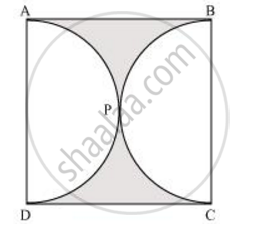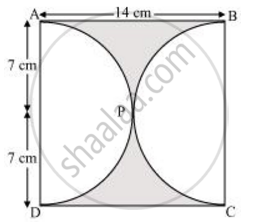# Find the area of the shaded region in the given figure, if ABCD is a square of side 14 cm and APD and BPC are semicircles. - Mathematics

Find the area of the shaded region in the given figure, if ABCD is a square of side 14 cm and APD and BPC are semicircles.  [Use Π = 22/7]#### Solution

It can be observed from the figure that the radius of each semi-circle is 7 cm.Area of each semi-circle = 1/2 pir^2

= 1/2xx22/7xx(7)^2

= 77 cm2

Area of square ABCD = (Side)2 = (14)2 = 196 cm2

= Area of square ABCD − Area of semi-circle APD − Area of semi-circle BPC

= 196 − 77 − 77 = 196 − 154 = 42 cm2

Concept: Areas of Combinations of Plane Figures
Is there an error in this question or solution?

#### APPEARS IN

NCERT Class 10 Maths
Chapter 12 Areas Related to Circles
Exercise 12.3 | Q 3 | Page 235